# Equations of Motion for a Particle (Part - 2) Civil Engineering (CE) Notes | EduRev

## Civil Engineering (CE) : Equations of Motion for a Particle (Part - 2) Civil Engineering (CE) Notes | EduRev

The document Equations of Motion for a Particle (Part - 2) Civil Engineering (CE) Notes | EduRev is a part of the Civil Engineering (CE) Course Introduction to Dynamics and Vibrations- Notes, Videos, MCQs.
All you need of Civil Engineering (CE) at this link: Civil Engineering (CE)

3.1.4 Velocity and acceleration in normal-tangential and cylindrical polar coordinates.

In some cases it is helpful to use special basis vectors to write down velocity and acceleration vectors, instead of a fixed {i,j,k} basis. If you see that this approach can be used to quickly solve a problem – go ahead and use it. If not, just use Cartesian coordinates – this will always work, and with MAPLE is not very hard. The only benefit of using the special coordinate systems is to save a couple of lines of rather tedious trigonometric algebra – which can be extremely helpful when solving an exam question, but is generally insignificant when solving a real problem.

Normal-tangential coordinates for particles moving along a prescribed planar path

In some problems, you might know the particle speed, and the x,y coordinates of the path (a car traveling along a road is a good example). In this case it is often easiest to use normal-tangential coordinates to describe forces and motion. For this purpose we

• Introduce two unit vectors n and t, with t pointing tangent to the path and n pointing normal to the path, towards the center of curvature
• Introduce the radius of curvature of the path R.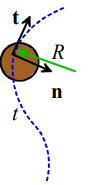If you happen to know the parametric equation of the path (i.e. the x,y coordinates are known in terms of some variable λ), then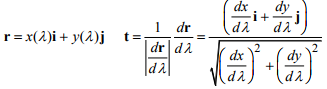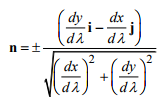The sign of n should be selected so that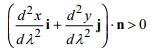The radius of curvature can be computed from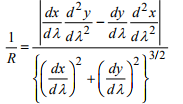The radius of curvature is always positive.

The direction of the velocity vector of a particle is tangent to its path. The magnitude of the velocity vector is equal to the speed.

The acceleration vector can be constructed by adding two components:

• the component of acceleration tangent to the particle’s path is equal dV / dt
• The component of acceleration perpendicular to the path (towards the center of curvature) is equal to V2 R/.

Mathematically v = Vt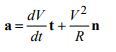Example: Design speed limit for a curvy road: As a consulting firm specializing in highway design, we have been asked to develop a design formula that can be used to calculate the speed limit for cars that travel along a curvy road.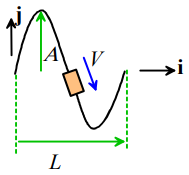The following procedure will be used:

• The curvy road will be approximated as a sine wave y = A sin(2π x / L) as shown in the figure - for a given road, engineers will measure values of A and L that fit the path. ·
• Vehicles will be assumed to travel at constant speed V around the path - your mission is to calculate the value of V ·
• For safety, the magnitude of the acceleration of the car at any point along the path must be less than 0.2g, where g is the gravitational acceleration. (Again, note that constant speed does not mean constant acceleration, because the cars direction is changing with time).

Our goal, then, is to calculate a formula for the magnitude of the acceleration in terms of V, A and L. The result can be used to deduce a formula for the speed limit

Calcluation:

We can solve this problem quickly using normal-tangential coordinates. Since the speed is constant, the acceleration vector is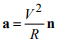The position vector is r = xi+ A sin(2π x / L) j , so we can calculate the radius of curvature from the formula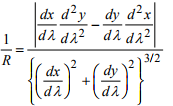Note that x acts as the parameter λ for this problem, and y = A sin (2πx / L) , so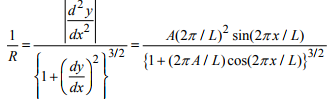and the acceleration is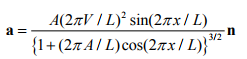We are interested in the magnitude of the acceleration…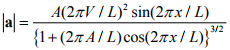We see from this that the car has the biggest acceleration when x = L / 2 . The maximum acceleration follows as

amax = A(2πV / L)2

The formula for the speed limit is thereforePolar coordinates for particles moving in a plane

When solving problems involving central forces (forces that attract particles towards a fixed point) it is often convenient to describe motion using polar coordinates.

Polar coordinates are related to x,y coordinates through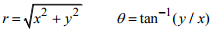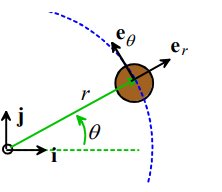Suppose that the position of a particle is specified by its ‘polar coordinates’ (r,θ) relative to a fixed origin, as shown in the figure. Let er be a unit vector pointing in the radial direction, and let eθ be a unit vector pointing in the tangential direction, i.e

er = cosθi + sinθj

eθ = -sin θi + cos θj

The velocity and acceleration of the particle can then be expressed as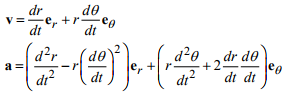Deriving these results takes some tedious algebra, but it’s conceptually simple – here’s what we do:

1. Write down the position vector in terms of (r,θ) in a fixed (i,j,k) coordinate system
2. Take the time derivatives to find acceleration and velocity in the (i,j,k) coordinate system
3. Convert the results to the {er,eθ,ez} coordinate system. To do this remember that the component of v parallel to er can be found using a dot product: vr = v.er . Similarly vθ = v.eθ

Here are the details with Mupad taking care of the tedious algebra.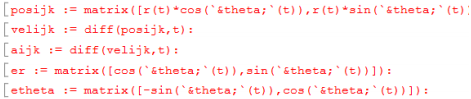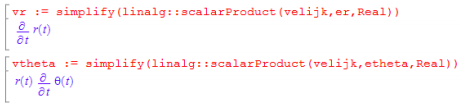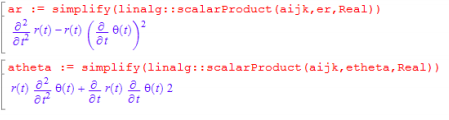Example The robotic manipulator shown in the figure rotates with constant angular speed ω about the k axis. Find a formula for the maximum allowable (constant) rate of extension dL / dt  if the acceleration of the gripper may not exceed g.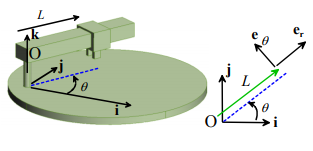We can simply write down the acceleration vector, using polar coordinates. We identify

ω = dθ / dt and r=L, so that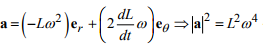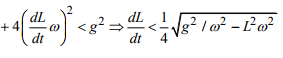Other examples using polar coordinates can be found in sections below.

3.1.5 Measuring position, velocity and acceleration

If you are designing a control system, you will need some way to detect the motion of the system you are trying to control.1. GPS – determines position on the earth’s surface by measuring the time for electromagnetic waves to travel from satellites in known positions in space to the sensor. Can be accurate down to cm distances, but the sensor needs to be left in position for a long time for this kind of accuracy. A few m is more common.

2. Optical or radio frequency position sensing – measure position by (a) monitoring deflection of laser beams off a target; or measuring the time for signals to travel from a set of radio emitters with known positions to the sensor. Precision can vary from cm accuracy down to light wavelengths.

3. Capacitative displacement sensing – determine position by measuring the capacitance between two parallel plates. The device needs to be physically connected to the object you are tracking and a reference point. Can only measure distances of mm or less, but precision can be down to micron accuracy.4. Electromagnetic displacement sensing – measures position by detecting electromagnetic fields between conducting coils, or coil/magnet combinations within the sensor. Needs to be physically connected to the object you are tracking and a reference point. Measures displacements of order cm down to microns.

5. Radar velocity sensing – measures velocity by detecting the change in frequency of electromagnetic waves reflected off the traveling object.

6. Inertial accelerometers: measure accelerations by detecting the deflection of a spring acting on a mass.

Accelerometers are also often used to construct an ‘inertial platform,’ which uses gyroscopes to maintain a fixed orientation in space, and has three accelerometers that can detect motion in three mutually perpendicular directions. These accelerations can then be integrated to determine the position. They are used in aircraft, marine applications, and space vehicles where GPS cannot be used.

3.1.6 Newton’s laws of motion for a particle

Newton’s laws for a particle are very simple. Let

1. m denote the mass of the particle
2. F denote the resultant force acting on the particle (as a vector)
3. a denote the acceleration of the particle (again, as a vector). Then

F = ma

Occasionally, we use a particle idealization to model systems which, strictly speaking, are not particles. These are:

1. A large mass, which moves without rotation (e.g. a car moving along a straight line)
2. A single particle which is attached to a rigid frame with negligible mass (e.g. a person on a bicycle)

In these cases it may be necessary to consider the moments acting on the mass (or frame) in order to calculate unknown reaction forces.

1. For a large mass which moves without rotation, the resultant moment of external forces about the center of mass must vanish.
2. For a particle attached to a massless frame, the resultant moment of external forces acting on the frame about the particle must vanish.

MC = 0

It is very important to take moments about the correct point in dynamics problems! Forgetting this is the most common reason to screw up a dynamics problem…

If you need to solve a problem where more than one particle is attached to a massless frame, you have to draw a separate free body diagram for each particle, and for the frame. The particles must obey Newton’s laws F = ma . The forces acting on the frame must obey F = 0 and MC = 0 , (because the frame has no mass).

The Newtonian Inertial Frame.

Newton’s laws are very familiar, and it is easy to write them down without much thought. They do have a flaw, however.

When we use Newton’s laws, we assume that we can identify a convenient origin somewhere that we regard as `fixed’. In addition, to write down an acceleration vector, we need to be able to choose a set of fixed directions in space.

For engineering calculations, this usually poses no difficulty. If we are solving problems involving terrestrial motion over short distances compared with the earth’s radius, we simply take a point on the earth’s surface as fixed, and take three directions relative to the earth’s surface to be fixed. If we are solving problems involving motion in space near the earth, or modeling weather, we take the center of the earth as a fixed point, (or for more complex calculations the center of the sun); and choose axes to have a fixed direction relative to nearby stars.

But in reality, an unambiguous inertial frame does not exist. We can only describe the relative motion of the mass in the universe, not its absolute motion. The general theory of relativity addresses this problem – and in doing so explains many small but noticeable discrepancies between the predictions of Newton’s laws and experiment.

It would be fun to cover the general theory of relativity in this course – but regrettably the mathematics needed to solve any realistic problem is horrendous. As engineers, we always have to solve realistic problems, and we usually can’t afford to spend a long time doing complicated calculations, so we use the simplest theory that will allow us to make the correct design decisions. Newton’s laws are fine for us…

Offer running on EduRev: Apply code STAYHOME200 to get INR 200 off on our premium plan EduRev Infinity!

## Introduction to Dynamics and Vibrations- Notes, Videos, MCQs

20 videos|53 docs

,

,

,

,

,

,

,

,

,

,

,

,

,

,

,

,

,

,

,

,

,

;